# Mega potion

This item is of drink class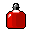You see a mega potion. It strengthens greatly.
Attributes
AMOUNT
800
FREQUENCY
1
REGEN
800
Dropped by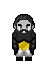duergar wizard
Drops between 1 and 2.
Probability: 10.5%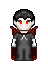vampire lord
Drops between 1 and 2.
Probability: 55%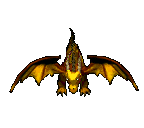flying golden dragon
Drops exactly 1.
Probability: 50%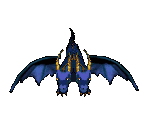twin headed dragon
Drops between 1 and 2.
Probability: 80%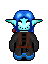dark elf matronmother
Drops between 1 and 2.
Probability: 50%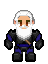blordrough general
Drops between 1 and 2.
Probability: 30%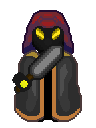black giant
Drops between 1 and 2.
Probability: 50%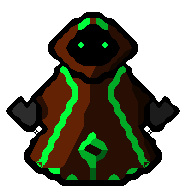necrosophia
Drops between 1 and 2.
Probability: 50%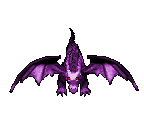rysin dragon
Drops between 1 and 2.
Probability: 80%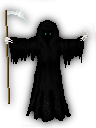black death
Drops between 2 and 4.
Probability: 50%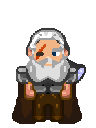Lord Durin
Drops between 1 and 4.
Probability: 20%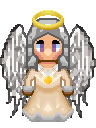archangel
Drops between 1 and 4.
Probability: 40%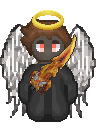dark angel
Drops between 2 and 4.
Probability: 50%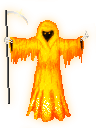golden death
Drops between 5 and 20.
Probability: 80%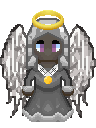dark archangel
Drops between 2 and 5.
Probability: 50%
Sold by